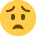# settlement discount

Hi everybody,

My exam is in 5 days and I feel unsure about the settlement discount when customer has paid within the period . EG
INVOICE with 2% settlement discount if paid within 7 days
net: 200
net 180
Vat 30.87 (180*2%=3.6 180-3.6*%7.5% 176.74*)
Total 210.87

and now I have two versions from two different teachers
if the customer pays within 2 days: 210.87*0.02=206.66
or 176.74+30.87 = 207.27

.

• I agree with your figures, £210.87.
Stick with what you are doing it looks good to me.
• Hi

If I work through:

Net = £200
After Discount Net = £180

VAT = £30.87

Calc: (180*0.98) * 0.175

Total = £210.87

• Surely you have already accounted for the settlement discount when you get £176.4, when you add the VAT 30.87, the total - 207.27- is the dicounted total, so no need to deduct the discount again?

• the problem is
1st teacher told me the answer was

210.87*2%=4.22 210.87- 4.22= 206.65 ( she said That was the answer)

2nd teacher a few days later:
176.40+30.87= 207.27 ( He said he was right the other teacher was wrong)

What should I answer to have the point at the aat level 2 exam?

Thank you for all your help
• Bloody hell, I thought I had this cracked before I read the other repliesIsn't this the answer if the discount IS taken:

180*0.98 = 176.4
176.4*0.175 = 30.87

176.4 + 30.87 = 207.27

?????????????????
• I agree with Matt, i get £207.27

200*0.10 = 20

180*0.02 = 3.60

200-20-3.60=176.40

176.40+ 30.87= £207.27 ???
• I think there may be confusion between what is on the invoice:
200-20=180
180 * 2% = 3.6.
180 -3.6 = 176.40 *17.5% = 30.87
Invoice total is 180 + 30.87 =210.87

and what customer actually pays:
210.87 less discount 3.6 = 207.27 but only if he pays within 7 days.
• jeanneldn wrote: »
and now I have two versions from two different teachers
if the customer pays within 2 days: 210.87*0.02=206.66
or 176.74+30.87 = 207.27
.

If you want to know what goes on the bottom of the invoice the answer will be £210.87 - like Cullen said - regardless of when the customer pays and weather or not he gets the settlement discount.

if he takes the discount, he'll pay only 207.27
• Correct all discounts are calculated before VAT is added, So the VAT should only be calculated on the Net price less 10% trade discount; ie £180 then you calculate the 2% early settlement dicount i.e £3.60 VAT is then calculated on the final net figure of £176.40.

The reason for settlement discounts being calculated before VAT is added is because it is assumed that the customer will always take the settlement discount.
• Settlement Discount

Hi

I agree with Matt and Nich - the answer is £207.27

Vat is always calculated on the discounted amount so you need to take the trade and settlement discounts off before calculating VAT and adding.
• thank you very much everybody I feel more confident now !!!!!!